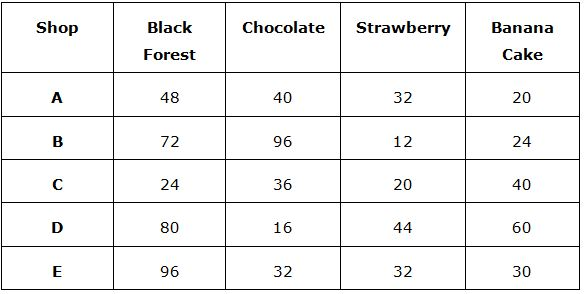# IBPS / LIC Clerk Prelims Quantitative Aptitude Questions 2019 (Day-03)

Dear Aspirants, Our IBPS Guide team is providing new series of Quantitative Aptitude Questions for IBPS Clerk Prelims 2019 so the aspirants can practice it on a daily basis. These questions are framed by our skilled experts after understanding your needs thoroughly. Aspirants can practice these new series questions daily to familiarize with the exact exam pattern and make your preparation effective.

Check here for IBPS Clerk Prelims Mock Test 2019

##### Check here for IBPS Clerk  Mock Test 2019
[WpProQuiz 7225]

wrong number series

Directions (01 – 05): Find out the wrong number in the following number series.

1) 9, 153, 274, 382, 455, 519

a) 153

b) 274

c) 519

d) 455

e) 382

2) 145, 134, 146, 133, 148, 132

a) 134

b) 146

c) 148

d) 133

e) 132

3) 21, 42, 70, 115, 147, 196

a) 42

b) 70

c) 147

d) 196

e) 115

4) 1, 513, 852, 1072, 1197, 1261

a) 1197

b) 1261

c) 1072

d) 852

e) 513

5) 9, 25, 45, 68, 97, 128

a) 97

b) 25

c) 128

d) 68

e) 45

Data Interpretation

Directions (6 – 10): Study the following information carefully and answer the questions given below.

The given table shows the amount of five different cake (in kg) sold by different shops.6) The amount of Chocolate cake sold in A and E together is approximately what percent of the amount of Banana Cake sold in C and D together?

a) 68%

b) 72%

c) 76%

d) 81%

e) None of these

7) What is the ratio of the amount of strawberry cake to Banana in all the shops together?

a) 71: 89

b) 4: 5

c) 69: 86

d) 70: 87

e) None of these

8) What is the difference between the amount of all the types of cake in B and D?

a) 2 kg

b) 4 kg

c) 6 kg

d) 8 kg

e) None of these

9) What is the average amount of black forest sold in all the shops together?

a) 64 kg

b) 62 kg

c) 60 kg

d) 58 kg

e) None of these

10) The amount of Strawberry cake sold in D is approximately what percent of the amount of all the types of cake sold in A?

a) 28%

b) 30%

c) 32%

d) 34%

e) 36%

Directions (1-5) :

9 + 122 = 153

153 + 112 = 274

274 + 102 = 374

374 + 92 = 455

455 + 82 = 519

The wrong term is 382

145 – 11 = 134

134 + 12 = 146

146 – 13 = 133

133 + 14 = 147

147 – 15 = 132

The wrong term is 148

21 + (3 * 7) = 42

42 + (4 * 7) = 70

70 + (5 * 7) = 105

105 + (6 * 7) = 147

147 + (7 * 7) = 196

The wrong term is 115

1 + 83 = 513

513 + 73 = 856

856 + 63 = 1072

1072 + 53 = 1197

1197 + 43 = 1261

The wrong term is 852

9 + 17 = 26

26 + 19 = 45

45 + 23 = 68

68 + 29 = 97

97 + 31 = 128

The wrong term is 25

Directions (6-10) :

Required percentage = [(40 + 32)/(40 + 60)] * 100 = 72%

Required ratio = (32 + 12 + 20 + 44 + 32):(20 + 24 + 60 + 40 + 30)

= 140: 174

=70: 87

B = 72 + 96 + 12 + 24 = 204

D = 80 + 16 + 44 + 60 = 200

Difference = 204 – 200 = 4

Required average = (48 + 72 + 24 + 80 + 96)/5 = 64